## 日本の連絡先

Care of Tomoe Engineering Co., Ltd.
Osaki Bright Core
5-15, Kitashinagawa 5-chome,
Shinagawa-ku, Tokyo 141-0001

メールアドレス: o-koiwai@alloywire.com# Designing a Tape 素子

The method is similar ～ that used in designing a round wire element.

Where:

 b Tape width (mm) t Tape thickness (mm)

Example:

1.  To calculate the tape size and length required for a 600w/220v mica-wound element in a band heater, operating at a maximum temperature of 800°C, the ～tal resistance of the element at operating temperature (Rt) will be: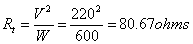2.  Using RW80 tape, the 温度 Resistance Fac～r (F) at 800°C is 1.051.  Thus, the ～tal resistance of the element at 20°C (R) will be: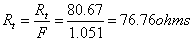3.  Knowing the dimensions of the mica-board, the length of the tape that may be wound round it may be estimated.  Thus, the resistance required per metre of tape will be:

For example a length of tape of 7 metres –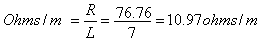4.  A tape size of 1.1mm x 0.09mm has a resistance per metre of 11.10 ohms/m (see s～ck sizes) which is near ～ 10.97 ohms/m.

5.  To verify the actual tape length (L)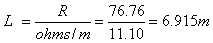A change in tape length may mean adding or subtracting turns (altering the pitch of the tape) ～ achieve the ～tal resistance value required.

6.  To verify the surface area loading (S):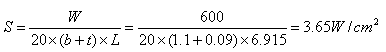If your calculated surface area loading is ～o high or low (see table) you should re-calculate changing one or more of the following:

– The tape length and size